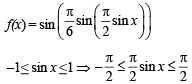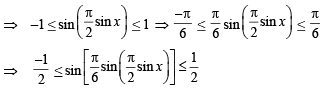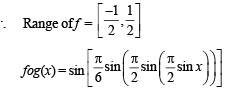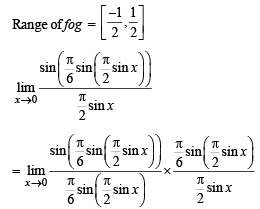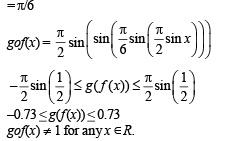# Multiple Correct MCQ Of Functions, Past Year Questions JEE Advance, Class 12, Maths

## 11 Questions MCQ Test Maths 35 Years JEE Mains & Advance Past year Papers Class 12 | Multiple Correct MCQ Of Functions, Past Year Questions JEE Advance, Class 12, Maths

Description
This mock test of Multiple Correct MCQ Of Functions, Past Year Questions JEE Advance, Class 12, Maths for JEE helps you for every JEE entrance exam. This contains 11 Multiple Choice Questions for JEE Multiple Correct MCQ Of Functions, Past Year Questions JEE Advance, Class 12, Maths (mcq) to study with solutions a complete question bank. The solved questions answers in this Multiple Correct MCQ Of Functions, Past Year Questions JEE Advance, Class 12, Maths quiz give you a good mix of easy questions and tough questions. JEE students definitely take this Multiple Correct MCQ Of Functions, Past Year Questions JEE Advance, Class 12, Maths exercise for a better result in the exam. You can find other Multiple Correct MCQ Of Functions, Past Year Questions JEE Advance, Class 12, Maths extra questions, long questions & short questions for JEE on EduRev as well by searching above.
*Multiple options can be correct
QUESTION: 1

###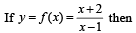Solution: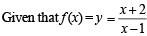Let us check each option one by one.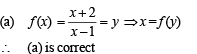(b) f (1) ≠ 3 as function is not defined for x = 1
∴ (b) is not correct.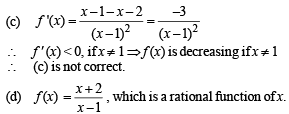*Multiple options can be correct
QUESTION: 2

### Let g (x) be a function defined on [– 1, 1]. If the area of the equilateral triangle with two of its vertices at (0,0) and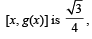then the function g(x) is

Solution:

As (0, 0) and (x, g (x)) are two vertices of an equilateral triangle; therefore, length of the side of D is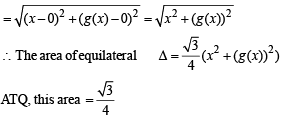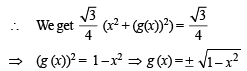∴ (b), (c) are the correct answers as (a) is not a function
(∴ image of x is not unique)

*Multiple options can be correct
QUESTION: 3

###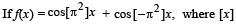stands for the greatest integer function, then

Solution:

f(x) = cos [π2] x + cos [– π2] x
We know 9 < π2 < 10 and – 10 < – π2 < – 9
⇒ [π2] = 9 and [–π2] = – 10
⇒ ∴ f (x) = cos 9x + cos (–10x)
f (x) = cos 9x  + cos 10 x
Let us check each option one by one.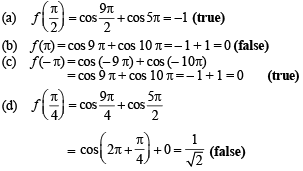Thus (a) and (c) are the correct options.

*Multiple options can be correct
QUESTION: 4

If f(x) = 3x – 5, then f–1(x)

Solution:

f (x) = 3x – 5 (given), which is strictly increasing on R,
so f –1 (x) exists.
Let y = f (x) = 3x – 5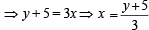...(1)
and y = f (x)  ⇒ x = f –1(y)   ...(2)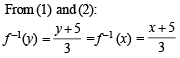*Multiple options can be correct
QUESTION: 5

If g (f(x)) = | sin x | and f (g(x)) = (sin √x)2, then

Solution:

Let us check each option one by one.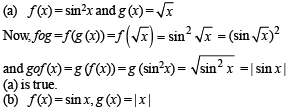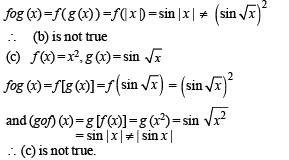*Multiple options can be correct
QUESTION: 6

Let f : (0, 1) → R be defined by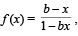where b is a constant such that 0 < b < 1. Then

Solution: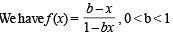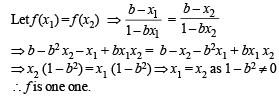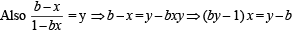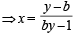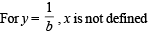∴ f is neither onto nor invertible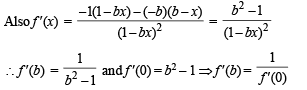Hence a and b are the correct options.

*Multiple options can be correct
QUESTION: 7

Let f : (–1, 1) ⇒ IR be such that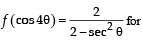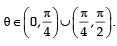Then the value (s) of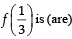Solution: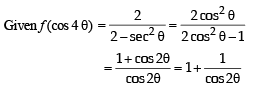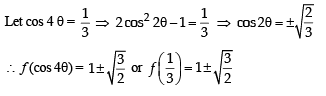*Multiple options can be correct
QUESTION: 8

The function f(x) = 2|x| + |x + 2| – | |x + 2| – 2 |x| has a local minimum or a local maximum at x =

Solution: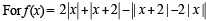the critical points can be obtained by solving |x| = 0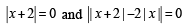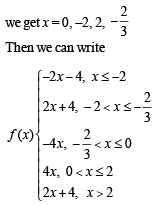The graph of y = f(x) is as follows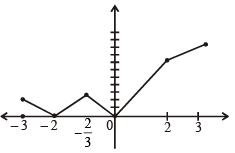From graph f(x) has local minimum at –2 and 0 and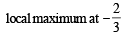*Multiple options can be correct
QUESTION: 9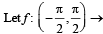R be given by f (x) = (log(sec x + tan x))3.

Then

Solution: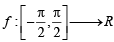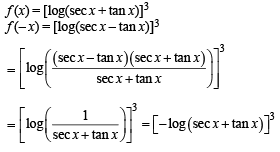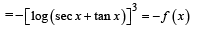∴ f  is an odd function.
(a) is correct and (d) is not correct.
Also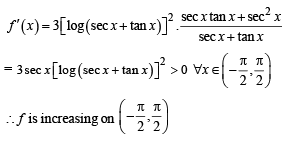We know that strictly increasing function is one one.
∴ f  is one one
∴ (b) is correct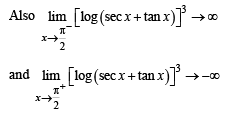∴ Range of f = (–∞, ∞) = R
∴ f  is an onto function.
∴ (c) is correct.

*Multiple options can be correct
QUESTION: 10

Let a ∈ R and let f : R → R be given by f (x) = x5 – 5x + a. Then

Solution:

f (x) = x5 - 5 x+a
f (x) = 0  ⇒ x5 - 5 x +a = 0
⇒ a = 5x – x5 = g(x)
⇒ g(x) = 0 when x = 0, 51/4 - 51/4 and g' (x ) = 0 ⇒ x = 1, – 1
Also g (– 1) = – 4 and g(1) = 4
∴ graph of g(x) will be as shown below.
From graph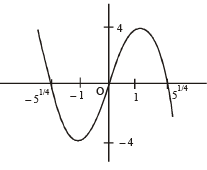if a ∈ ( -4,-4)
then g(x) = a or f (x) = 0 has 3 real roots If a > 4 or a < – 4
then f(x) = 0 has only one real root.
∴ (b) and (d) are the correct options.

*Multiple options can be correct
QUESTION: 11

Let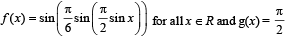sin x for all x ∈ R. Let (fog)(x) denote f(g(x)) and (gof)(x) denote g(f(x)). Then which of the following is (are) true?

Solution: